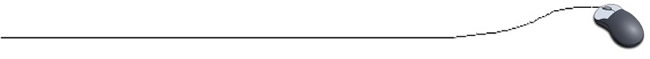Working with Strings Although = = does correctly test two values, such as two numbers, to see if they are equal, it has a different meaning when applied to strings. All strings are in the String class, so = = applied to two strings does not test to see whether the strings are equal.  To test two strings to see if they have equal values, you must use the method equals rather than = =. s1.equals(s2)  checks to see if string s1 is equal to string s2. The expressions s1.equals(s2) and  s2.equals(s1) are equivalent.   The method equalsIgnoreCase behaves similarly to equals, except that with equalsIgnoreCase the uppercase and lowercase versions of the same letter are considered the same.  Wally and wally would be considered equal. if ("Wally".equalsIgnoreCase("wally")) System.out.println("The same."); //This code prints "The same."